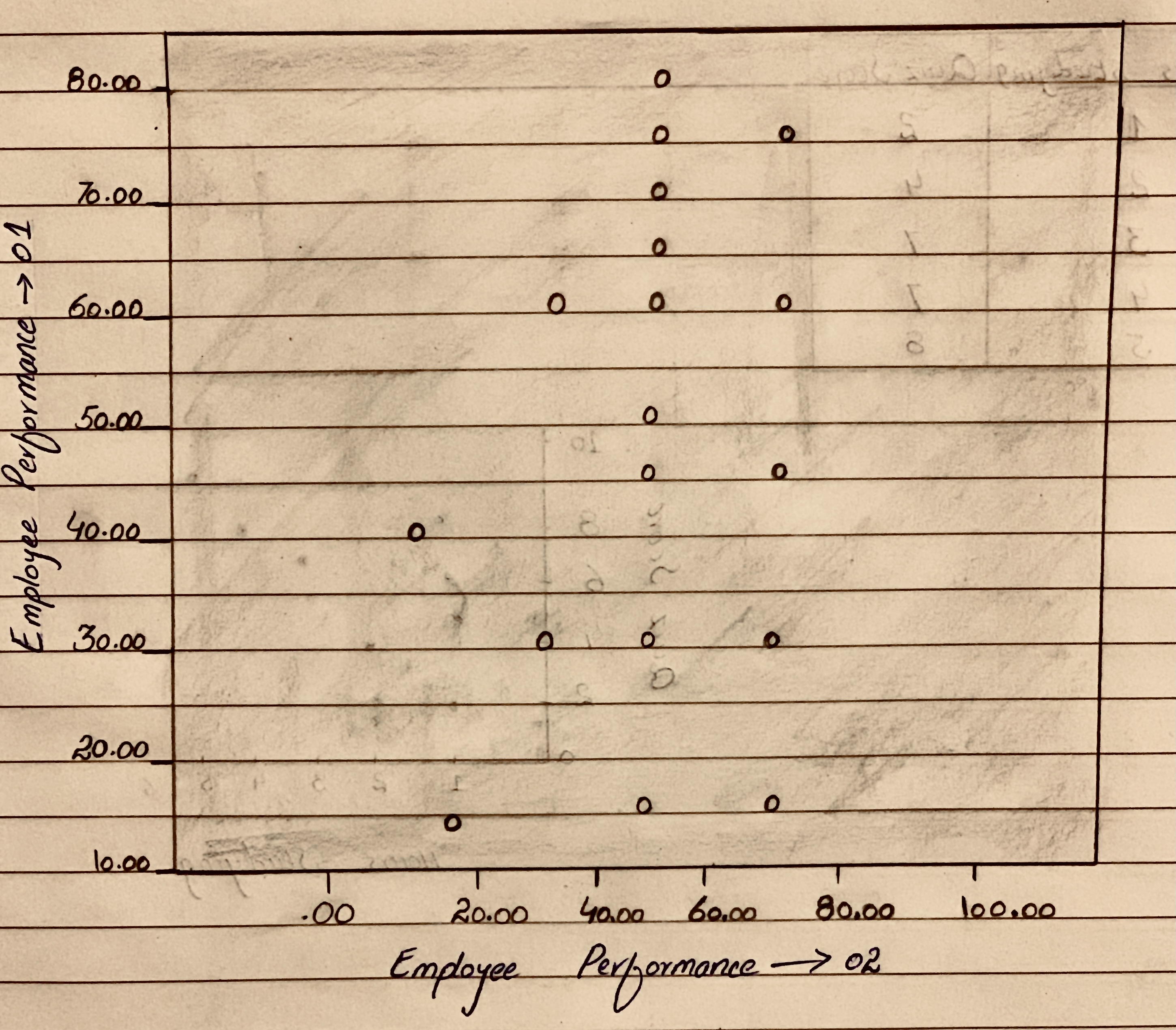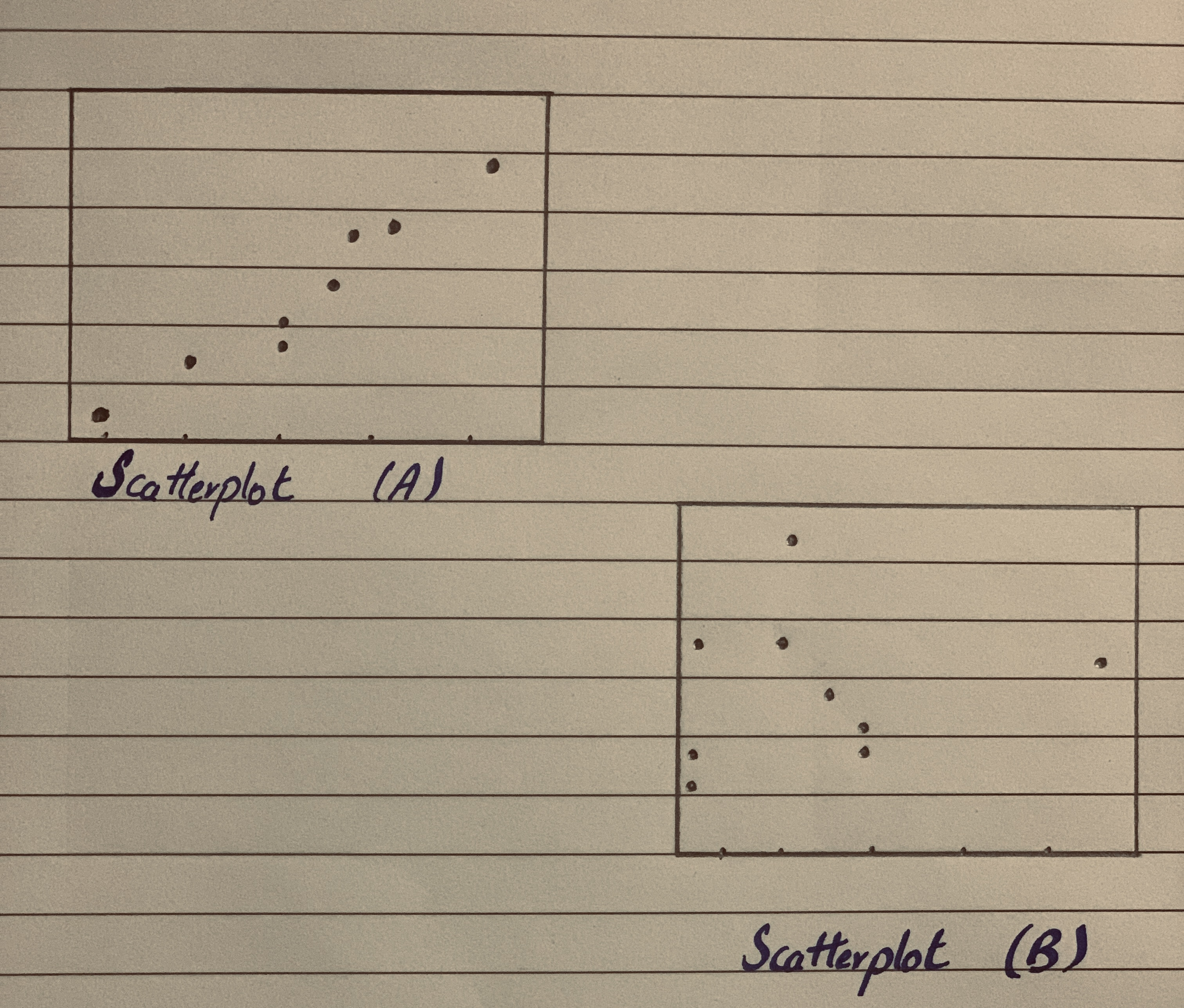">

# Matching Here are several scatterplots. The calculated correlations are -0.923, -0.487, 0.006, and 0.777. Which is which?Question
ScatterplotsMatching Here are several scatterplots. The calculated correlations are -0.923, -0.487, 0.006, and 0.777.
Which is which?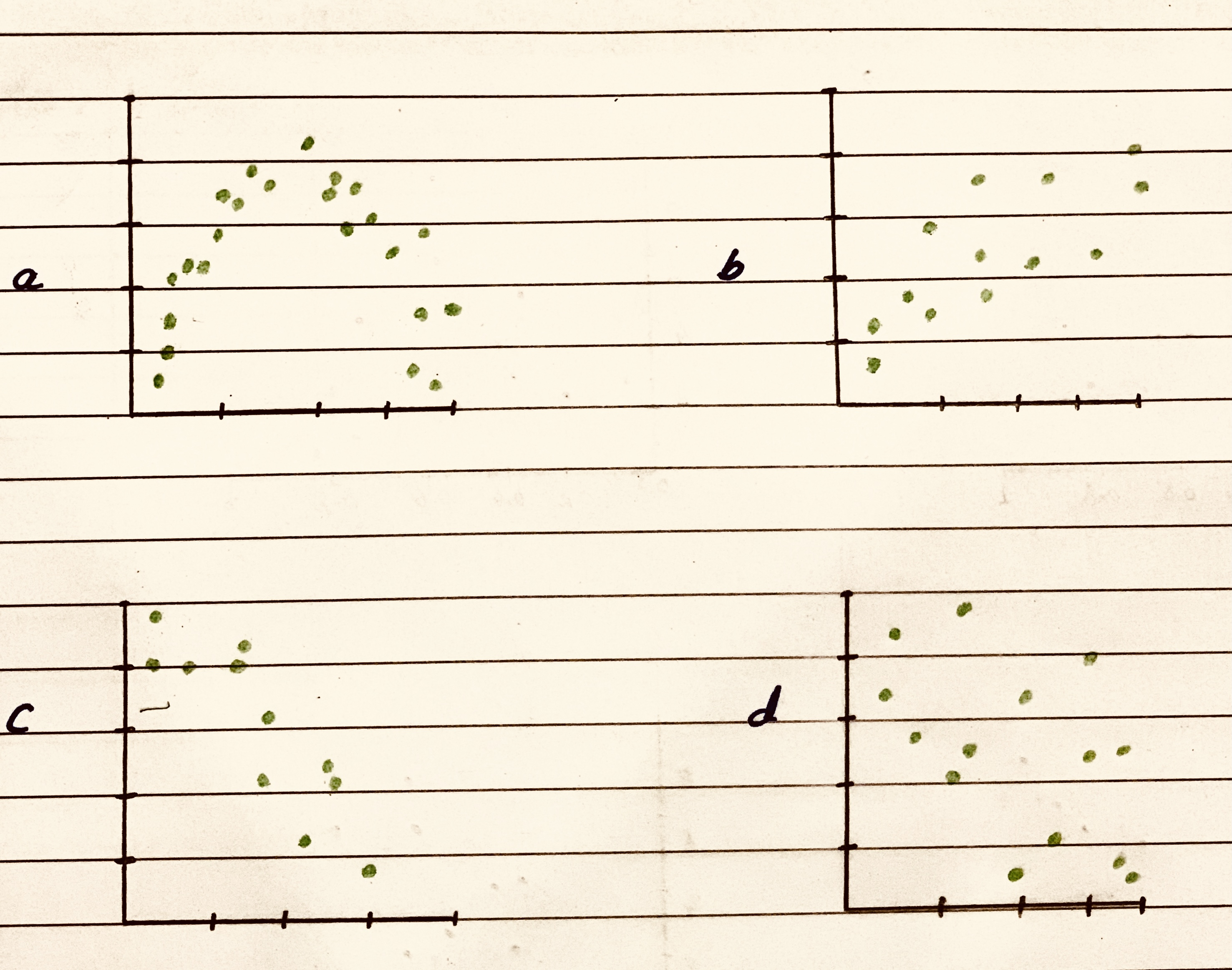2020-10-24
The scatter plots gives the graph of the two variables from which one can depict the relationship between the two variables.
The correlation is a measure which tells mathematically the kind of relationship the two variables has. It can be a direct, inverse, or no relationship.
The scatter plot (a) depicts that initially, as the value of one variable increases, the value of other variable also increases and then the pattern is changed, now as the value of one variable increases, the value of other variable also decreases.
But it can be seen that there is significant dip in the values of the variable when the other variables' value increases. Hence, overall it must have negative correlation but a moderate negative correlation.
Therefore, it must have correlation of -0.487.
The scatter plot (b) depicts that overall as the value of one variable increases, the value of other variable also increases. It can be seen that there is significant rise in value of the variable when other increases.
Hence, it must have strong positive correlation.
Therefore, it must have correlation of 0.777.
The scatter plot (c) depicts that overall as the value of one variable increases, the value of other variable also decreases and it can be seen that the data points are very close to each other.
Hence, it must have strong negative correlation.
Therefore, it must have correlation of -0.923.
The scatter plot (d) depicts that overall there is no pattern in which they are moving forward. Hence, the correlation must be very close to zero.
But it can be seen that there are more data points where the value of both the variables have increased.
Therefore, it must have correlation of 0.006.

### Relevant QuestionsStraight Lines Graph the straight lines in Exercises 1–3.
Then find the change in y for a one-unit change in x, find the point at which the line crosses the y-axis, and calculate the value of y when x 52.5.
1. y x 5 1 2.0 0.5
2. y x 5 1 40 36.2
3. y x 5 25 6
Scatterplots For the scatterplots in Exercises 5 describe the pattern that you see. How strong is the pattern? Do you see any outliers or clusters?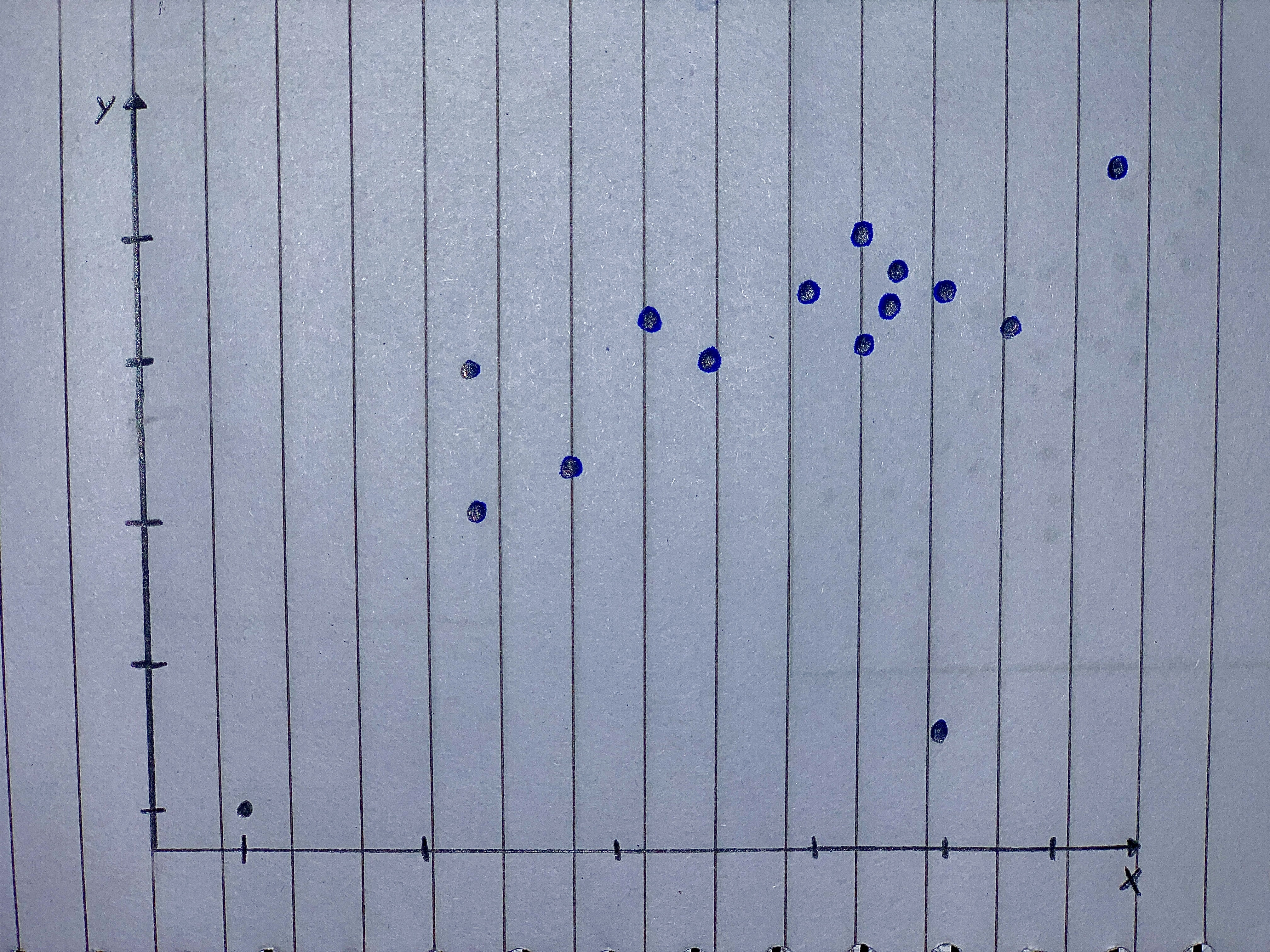Match the values of r with the accompanying scatterplots 0.723,-0.353,0.353,0.998 and -0.998
scatterplots 1,2,3,4,5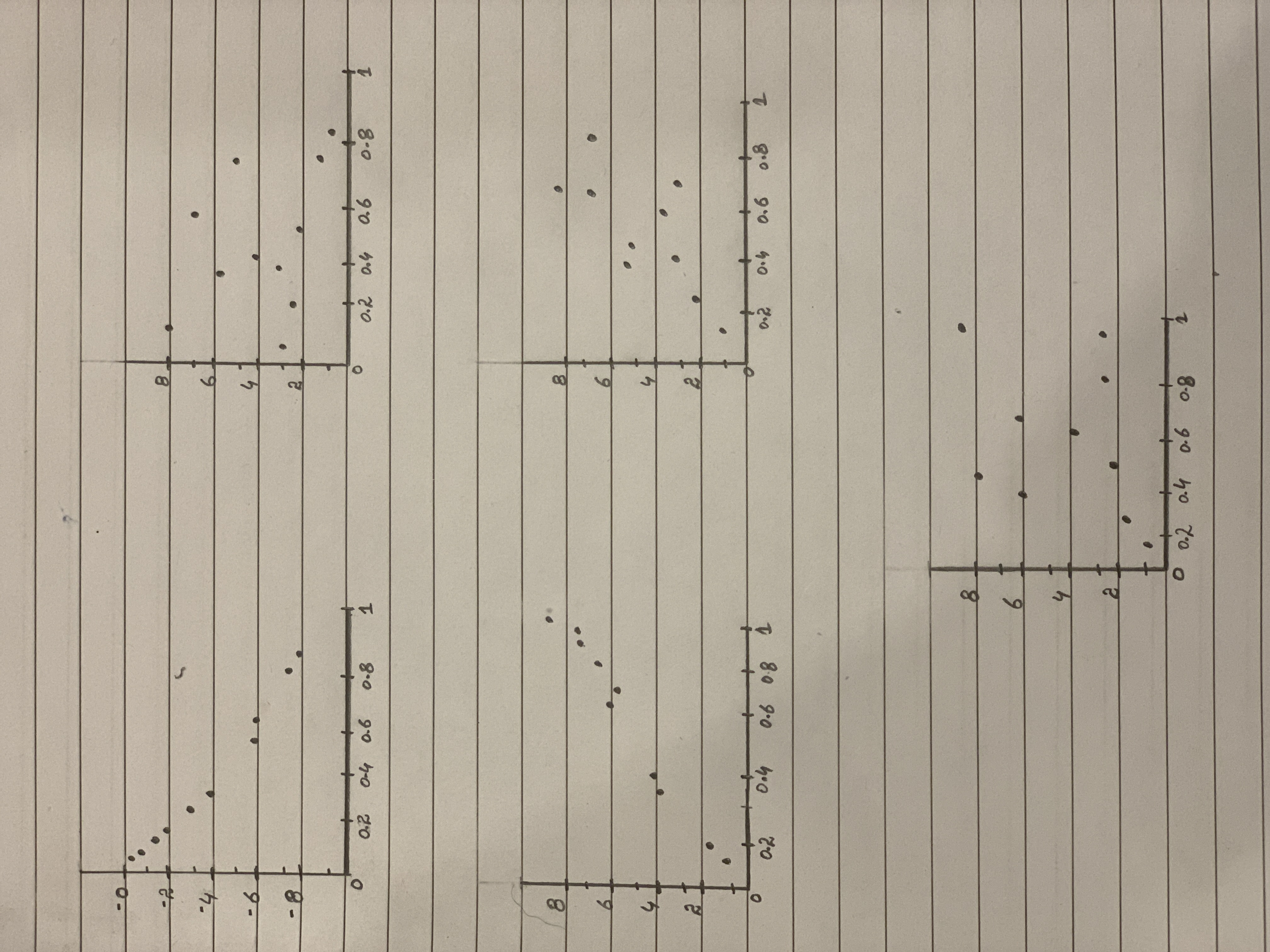Scatterplots Which of the scatterplots below show
a) little or no association?
b) a negative association?
c) a linear association?
d) a moderately strong association?
e) a very strong association?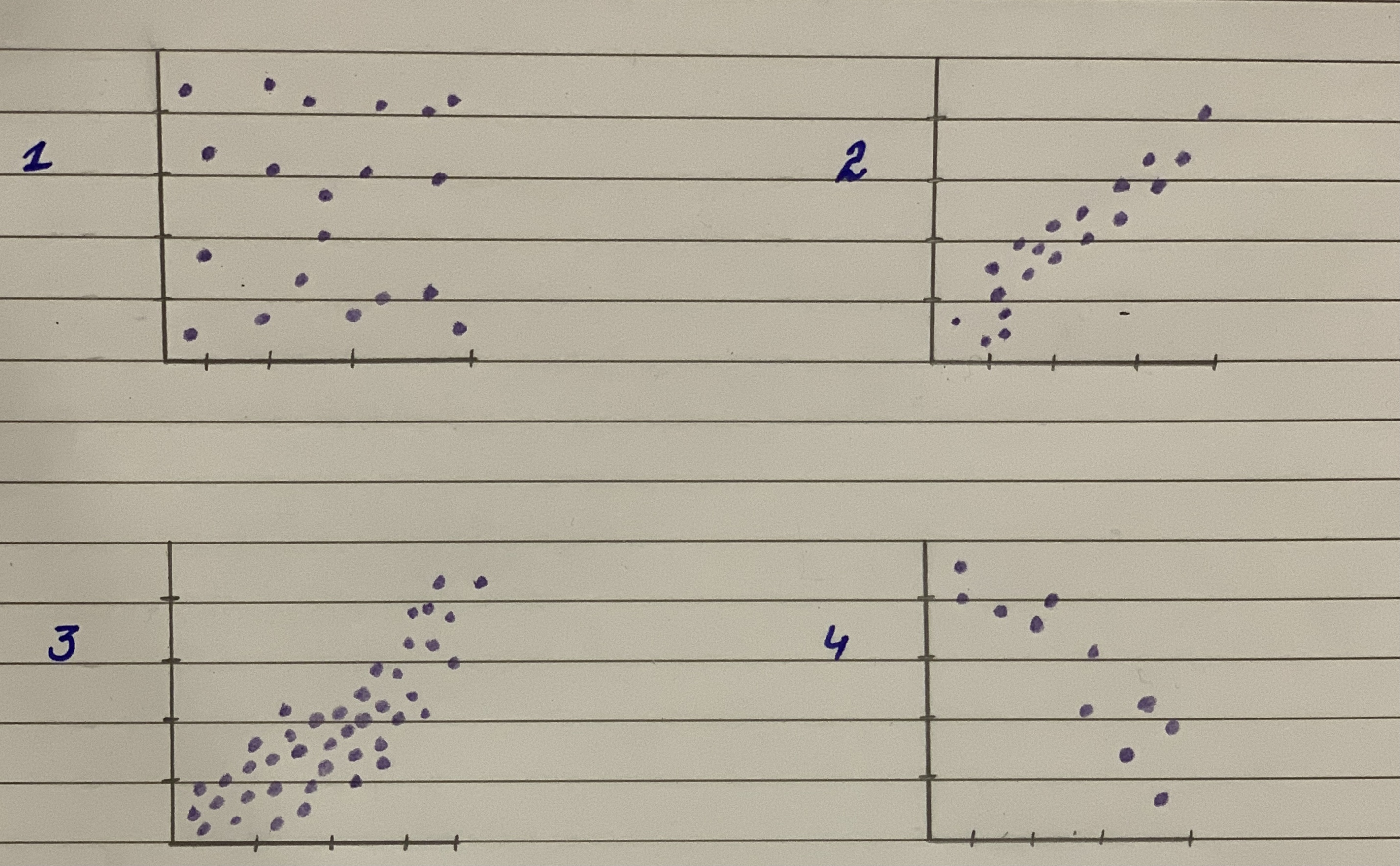Unusual points Each of the four scatterplots that follow shows a cluster of points and one “stray” point. For each, answer these questions:
1) In what way is the point unusual? Does it have high leverage, a large residual, or both?
2) Do you think that point is an influential point?
3) If that point were removed, would the correlation be- come stronger or weaker? Explain.
4) If that point were removed, would the slope of the re- gression line increase or decrease? Explain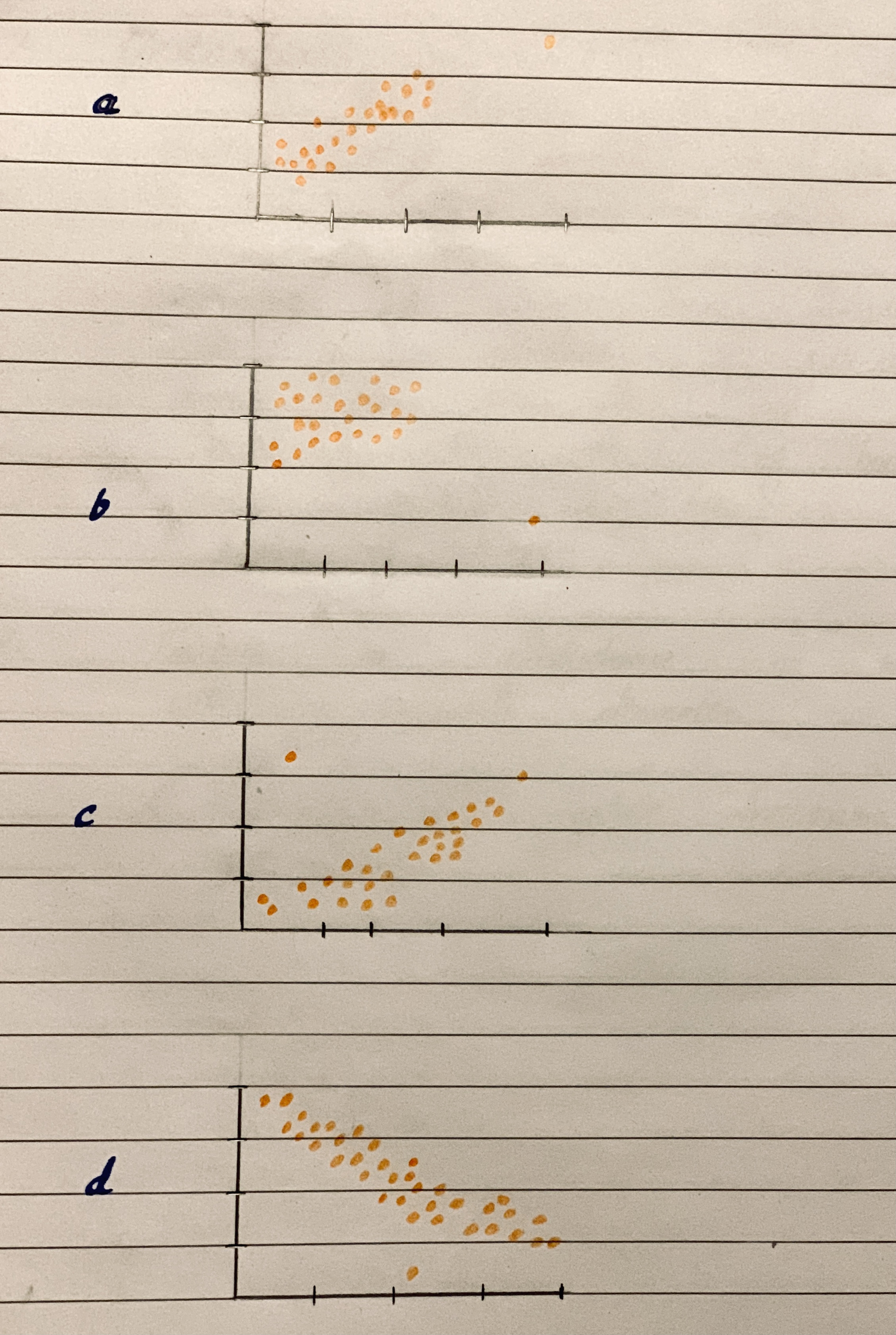Horace keeps track of the amount of time he studies and the score he gets on his quizzes. The data are shown in the table below. Which of the scatter plots below accurately records the data?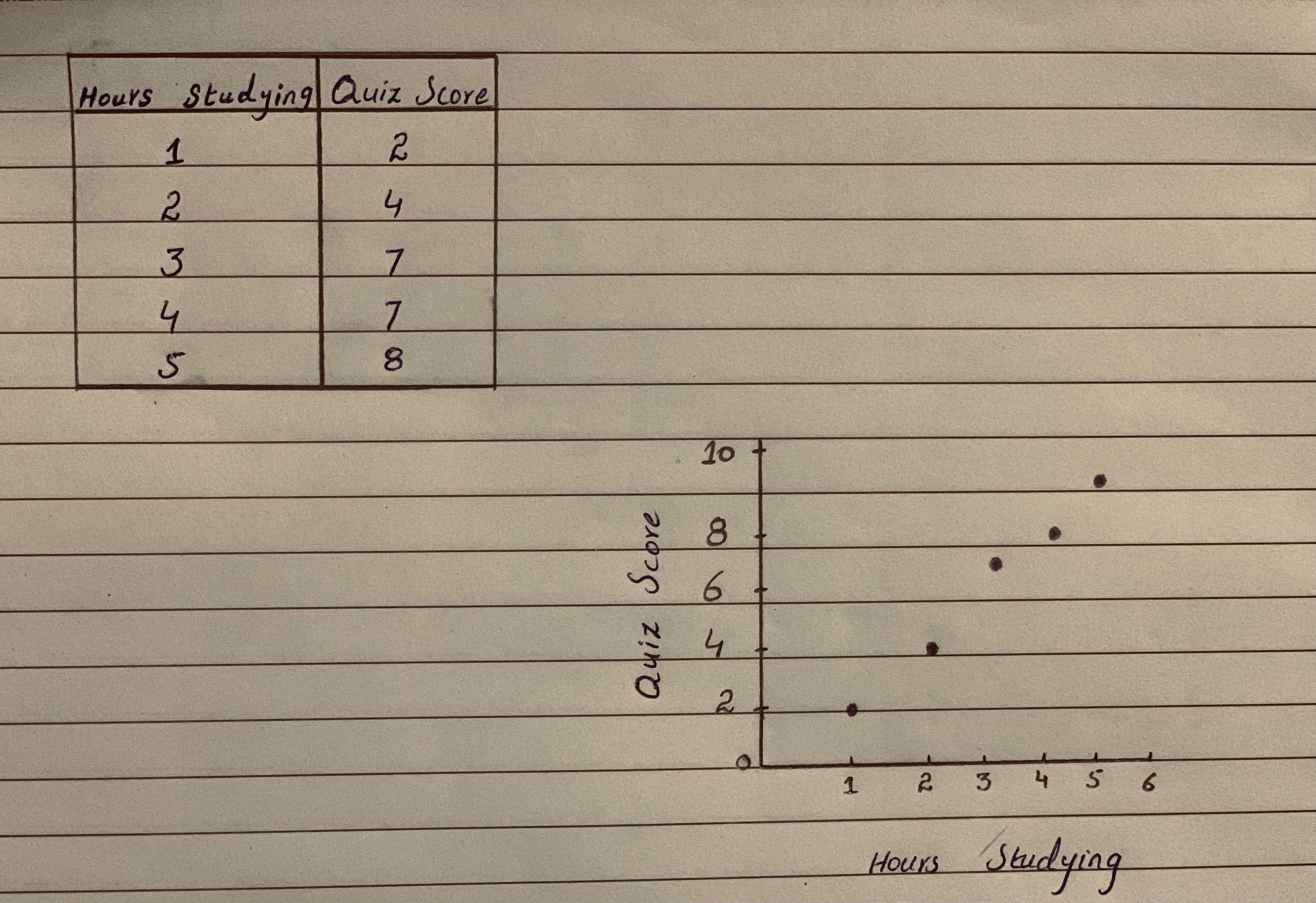Predicting Land Value Both figures concern the assessed value of land (with homes on the land), and both use the same data set. (a). Which do you think has a stronger relationship with value of the land-the number of acres of land or the number of rooms n the homes? Why? b. Il you were trying to predict the value of a parcel of land in e arca (on which there is a home), would you be able to te a better prediction by knowing the acreage or the num- ber of rooms in the house? Explain. (Source: Minitab File, Student 12. "Assess.")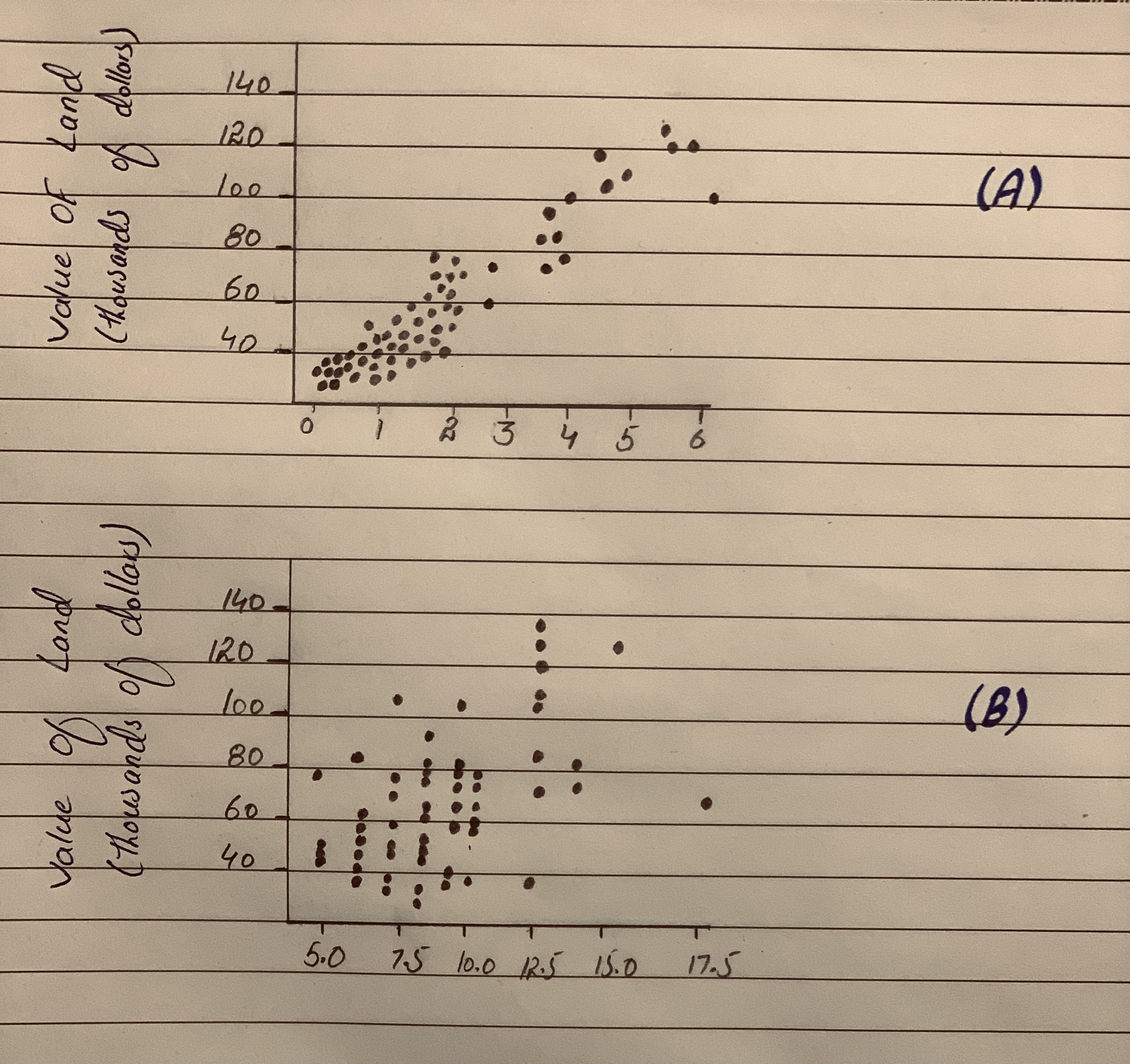Which two of the four scatter plots have most positive correlation and closer to zero correlation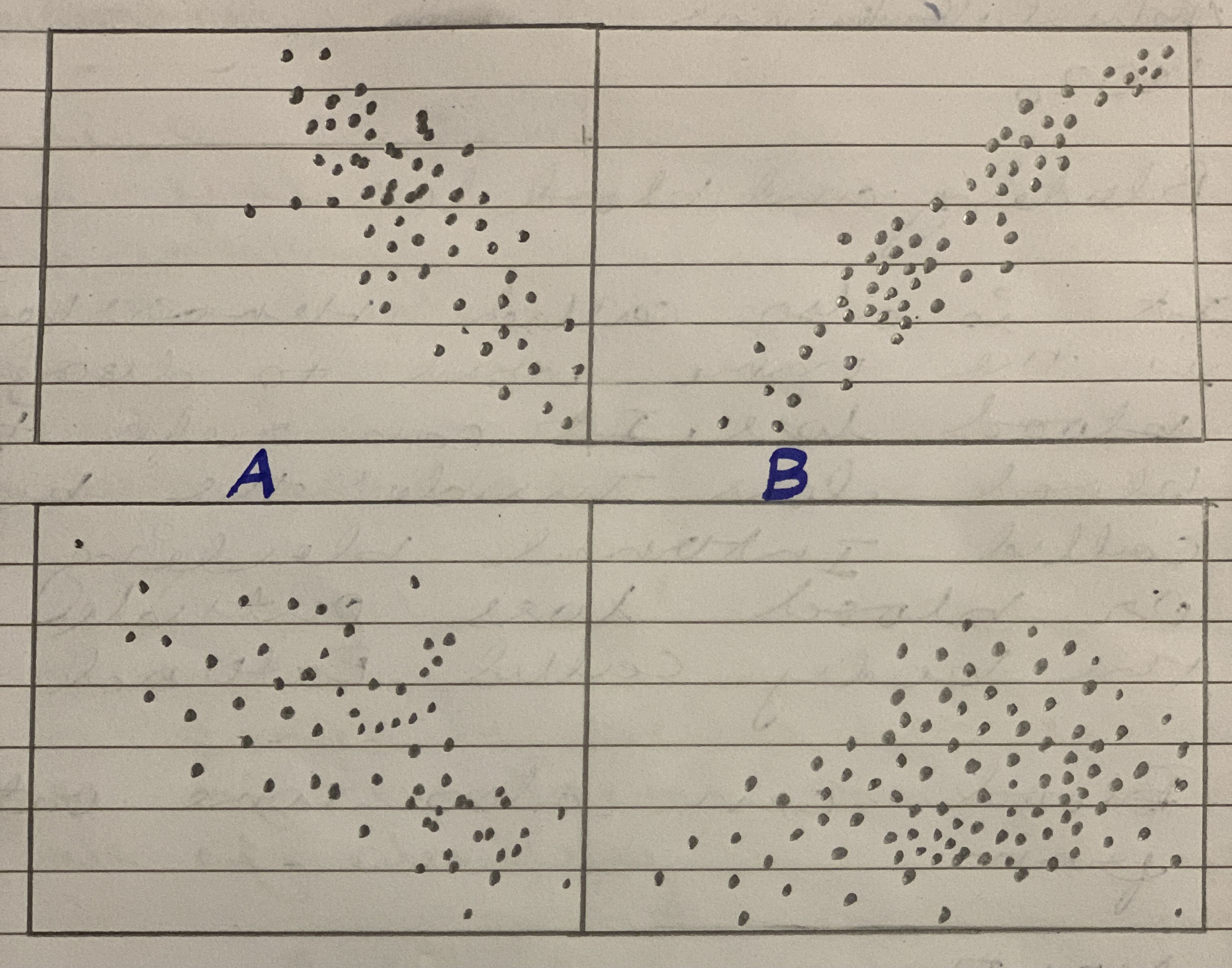We have to explain the relationship between employee performance 01 and employee performance 02 using the scater plot